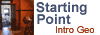# Sea Floor Spreading IThis material was originally created for Starting Point:Introductory Geology
and is replicated here as part of the SERC Pedagogic Service.

#### Summary

In this introductory Excel tutorial, students use Excel to explore the geodynamics Model equation for ocean depth around a sea-floor spreading center (see David T Sandwell or Turcotte and Schubert, 2002). The activity is primarily an introductory tutorial on Excel for students with no prior Excel experience. The use of the equation relating ocean floor depth to sea floor spreading rate and distance from spreading center provides a geoscience context. Activity II (see teaching notes and tips below) is a follow-up activity that has students use Excel to graphically compare the model predictions to observations from several North Atlantic cross-sections.

Brief overview of Ocean Depth/Sea Floor Spreading Model

Used this activity? Share your experiences and modifications

## Learning Goals

Students are carefully guided through using Excel to:
• input numbers and labels, format fonts, numbers, alignment, and cell widths
• use simple equations and copy these to other cells to generate a table from a mathematical formula (analytical model)
• learn about fixed and variable cell references in Excel equations
• make and format graphs with multiple curves
• learn how ocean topography is predicted (i.e. modeled) to vary with sea floor spreading rates, mantle density and mantle temperatures.

## Context for Use

Introductory course in Earth Science in which you want students to gain familiarity with Excel and for which sea floor spreading is an appropriate course topic.

## Description and Teaching Materials

We provide the following resources with this module:

• Activity Sheet (Acrobat (PDF) 550kB Sep8 03)
• Answers Document (Excel 37kB Apr3 04) with several sheets representing different levels of completion. These Excel documents can be used for the following purposes:
• Introducing concepts and ideas in an interactive lecture.
• Making transparencies or Power Point slides.
• Checking student results.

## Teaching Notes and Tips

This activity will take students about two hours to complete. Students should be able to work through this activity at home or in a computer lab will no supervision. Students more familiar with Excel can work through this activity more quickly.

In a follow-up activity (Sea Floor Spreading II), students compare actual data from Topex satellite measurements to the model predictions of how sea floor spreading rates influence ocean topography. North Atlantic cross-sections at 30° N, 40° N , and 50° N latitude are provided as is information on how to retrieve Topex elevation data from any location globally.

## Assessment

Grading the completed activity sheet provided can be used as an assessment of student understanding. The future success of students on other Excel related activities is also another useful assessment tool.

A link to an Excel Answers Document is provided above with several sheets representing different levels of completion for this activity. This can help instructors:

• assess student success for this acivity;
• answer student questions that come up;
• quickly familiarize themselves with the activity.
Below we show sample images from this Excel Answer Document.

Figure 1. Predicted (modeled) ocean topography for three different spreading rates, U.

Figure 2. Predicted ocean topography for C=0.11, 0.10, and 0.15 (Exp 1,2, and 3) corresponding to base values of mantle density and temperature of:
3.3 g/cc and 1580 K Exp 1 (control)
4.3 g/cc and 1580 K Exp 2 (30% increase in mantle density)
3.3 g/cc and 2050 K Exp 3 (30% increase in mantle temperature)

{See Brief overview of Ocean Depth/Sea Floor Spreading Model for a description of the model equation, c, and how it relates to mantle temperature and density.}

## References and Resources